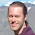## Saturday, March 3, 2012

### Prediction of cyclin-dependent kinase 2 inhibitor potency using the fragment molecular orbital method

M. P. Mazanetz, O. Ichihara, R. J. Law, M. Whittaker Journal of Cheminformatics 2011, 3:2 (Open Access)

The paper describes a surprisingly accurate method for predicting ligand-protein binding energies based, in part, on MP2/6-31G(d) ligand-protein interactions computed using the Fragment Molecular Orbital (FMO) method.  The correlation between predicted and observed binding free energies are $r^2=0.939$ and $r^2=0.842$ for the training and testing set, respectively.  The method is sufficiently efficient to be used within an industrial setting.  The method performs better than more conventional scoring function implemented in the MOE program.

The binding free energy is given by$$\Delta G_{bind}=X_H \Delta H_{bind}+X_{TS} (T \Delta S_{bind})+X_{psolv} \Delta\Delta G_{psolv}+X_{npsolv} \Delta\Delta G_{npsolv}+X_{residual}$$
$\Delta H_{bind}$ is the ligand-protein interaction energy computed from an FMO energy decomposition analysis using a small structural model of the protein. $T\Delta S_{bind}$ is set to 1 kcal/mol per rotateable bond, $\Delta\Delta G_{psolv}$ and $\Delta\Delta G_{npsolv}$ are computed using the Poisson-Boltzmann equation and the solvent accessible surface area, respectively.

The four coefficients are obtained using a partial least squares to latent structures analysis on 14 ligand-protein complexes (for which x-ray coordinates were available).  Surprisingly, $X_{TS}$ came out negative, so it is doubtful whether this term actually reports on an entropy change. The method is then applied to a test set of 14 new ligands for which x-ray structures where not available.

Another interesting feature of the method is that only the hydrogen atoms of the x-ray structures were geometry optimized (using MMFF94x).  In the case where x-ray structures were not available, the structures of the ligand-protein complexes "were built by manually modifying the reference ligand and then energy minimising the ligand whilst keeping the reference heavy atoms fixed".

Results where  $\Delta H_{bind}$ is computed using the MMFF94x force field instead of FMO was not reported.  This would answer the question whether FMO is truly needed.

Acknowledgments: I thank Dmitri Fedorov for alerting me to this paper, and Michael Mazanetz for helpful discussions.  Note that in Table 2 the column labels for the experimental and computational binding free energies should be switched.

Update (4/3/2012): $X_H=0.018613, X_{TS}=-0.485403, X_{psolv}=-0.024463, X_{npsolv}=1.729698, X_{residual}=0.258$.  The latter parameter is in kcal/mol.1.Nice paper.However, as always, it would have been nice to see a comparison to cheaper QM based methods. FMO based on MP2 must require some serious CPU time, and on some data I've found that dispersion corrected DFT calculations can give good results on active site models in ca. 15-30 minutes on 8 cpus.

1.One of the limitations with DFT is of course the lack of a dispersion energy term.

2.Dear anonymous, that's why I wrote "dispersion corrected DFT".

2.FMO using small systems (500 atoms) only takes 0.5-2hs to run on 16 CPUs. Thus making it an attractive method for tracking SAR and supporting medicinal chemistry efforts. Hence 10s of compounds can be processed in a day adding real value to drug discovery
FMO is great as it offers pair interaction energy decomposition analysis, thus a thorough understanding of the QM contributions giving rise to the interaction energies can be gained. This is important when considering non-classical interactions between a ligand and a receptor.

1.Still, what is gained by using MP2 compared to less computationally demanding methods? FMO might as well be implemented using cheaper QM methods such as dispersion corrected DFT or even semi-empirical PM6 with dispersion correction. Then it would be much faster, and the big question is of course what the loss of accuracy would be?

2.Electron correlation, treating CT effectively.

3.It's a very nice result, but it's still a bit frustrating that one cannot get the good binding energies out of the box as a result of FMO!

Also, it would have been interesting to see the fitting coefficients in the paper, to see which energies give most contribution to the fit.

1.Yes, but it's a very tough problem to get right. Especially the non-polar part of the desolvation energy.

Yes, strange that they weren't included to begin with, but Mike Mazanetz readily agreed to have them published in CCH.